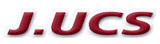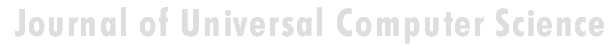Search
 Submission Procedure share: |available in:   PDF (212 kB) PS (1 MB)

get:
 Similar Docs BibTeX Write a comment
get:

DOI:   10.3217/jucs-020-09-1259

### On Alternative Approaches for Approximating the Transposition Distance

Gustavo Rodrigues Galvão (University of Campinas, Brazil)

Zanoni Dias (University of Campinas, Brazil)

Abstract: We study the problem of sorting by transpositions, which consists in computing the minimum number of transpositions required to sort a permutation. This problem is NP-hard and the best approximation algorithms for solving it are based on a standard tool for attacking problems of this kind, the cycle graph. In an attempt to bypass it, some researches posed alternative approaches. In this paper, we address three algorithms yielded by such approaches: a 2.25-approximation algorithm based on breakpoint diagrams, a 3-approximation algorithm based on permutation codes, and a heuristic based on longest increasing subsequences. Regarding the 2.25-approximation algorithm, we show that previous experimental data on its approximation ratio are incorrect. Regarding the 3-approximation algorithm, we close a missing gap on the proof of its approximation ratio and we show a way to run it in O(n log n) time. Regarding the heuristic, we propose a minor adaptation that allow us to prove an approximation bound of 3. We present experimental data obtained by running these algorithms for all permutations with up to 13 elements and by running these algorithms and the best known algorithms based on the cycle graph for large permutations. The data indicate that the 2.25-approximation algorithm is the best of the algorithms based on alternative approaches and that it is the only one comparable to the algorithms based on the cycle graph.

Keywords: approximation algorithms, genome rearrangement, sorting by transpositions

Categories: F.2.0, G.2.3